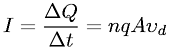Equations > Physics > Electricity and Magnetism > Electric current

### Electric currentLatex Code:

MathML Code:

 $I=\frac\Delta Q\Delta t=\mathrm{nqA}{\upsilon }_{d}$

MathType 5.0: